#Essential Math for Data Science:  ‘Why’ and ‘How’

It always pays to know the machinery under the hood (even at a high level) than being just the guy behind the wheel with no knowledge about the car.

Mathematics is the bedrock of any contemporary discipline of science. It is no surprise then that, almost all the techniques of modern data science (including all of the machine learning) have some deep mathematical underpinning or the other. In this article, we discuss the essential math topics to master to become a better data scientist in all aspects.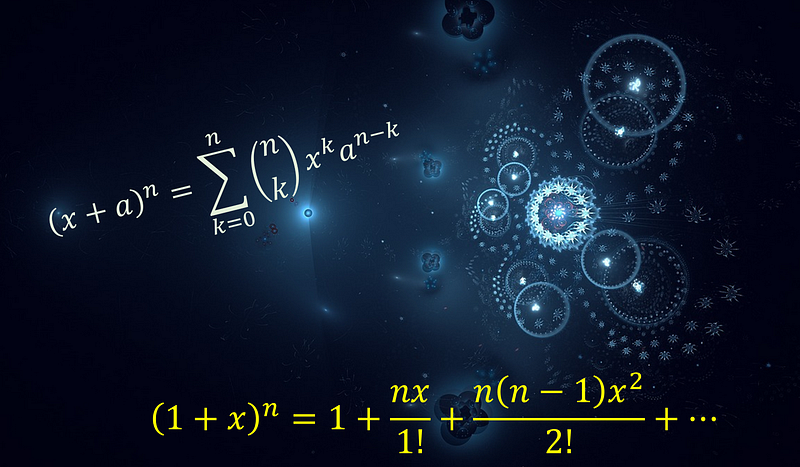### Introduction

Mathematics is the bedrock of any contemporary discipline of science. It is no surprise then that, almost all the techniques of modern data science (including all of the machine learning) have some deep mathematical underpinning or the other.

Sometimes, as a data scientist (or even as a junior analyst on the team), you have to learn those foundational mathematics by heart to use or apply the techniques properly, other times you can just get by using an API or the out-of-box algorithm.

However, having a solid understanding of the math behind the cool algorithm you are using to create meaningful product recommendation for your users, will never hurt you. More often than not, it should give you an edge among your peers and make you more confident. It always pays to know the machinery under the hood (even at a high level) than being just the guy behind the wheel with no knowledge about the car.

It goes without saying that you will absolutely need all the other pearls of knowledge, programming ability, some amount of business acumen, and your unique analytical and inquisitive mindset about the data to function as a top data scientist. All I am trying to do is to gather the pointers to the most essential math skills to help you in this endeavor.

### Of particular importance to ‘newcomers’

The knowledge of the essential math is particularly important for professionals who are trying to get into this field after spending a significant amount of time in some other domain — hardware engineering, retail, chemical process industry, medicine and health care, business management, etc…

Although one may think that (s)he has worked enough with spreadsheets and numerical calculations and projections in her current job, the demand of necessary math skill is significantly different in the practice of data science.

### Why and how is it different — It’s the SCIENCE not the DATA

Consider a web developer(or a business analyst). (S)he may be dealing with lot of data and information on a daily basis but there may not be an emphasis on rigorous modeling of that data. Often, there is immense time pressure, and the emphasis is on ‘use the data for your immediate need and move on’ rather than on deep probing and scientific exploration of the same. Whether you like it or not, data science should always be about the science (not data), and following that thread, certain tools and techniques become indispensable. Most of them are the hallmarks of sound scientific process,

• Modeling a process (physical or informational) by probing the underlying dynamics,
• Constructing hypotheses,
• Rigorously estimating the quality of the data source,
• Quantifying the uncertainty around the data and predictions,
• Training one’s sense for identification of the hidden pattern from the stream of information,
• Understanding clearly the limitation of a model
• (Occasionally) trying to understand a mathematical proof and all the abstract logic behind it

This kind of training, much of it— ability to think not in term of dry numbers but abstract mathematical entities (and their properties and inter-relationships), is imparted as part of standard curriculum of a four-year college level science degree program. One does not need to be a summa cum laude from a top university to have past access to this kind of mathematics, but unfortunately, that past access pretty much languishes at that point of the road and often does not get carried forward in our mental processes :-)

And, I am not talking about that differential calculus course back in the freshman year. I am thinking something simpler… like the number 2…

Say you are setting in your desk in the morning — all fresh and ready to tackle complex business charts for the day. Suddenly an email from your boss (or the mathematically minded friend) with this challenge— “Produce a proof in 2 minutes that square root of 2 is a not a rational number.”

Wait… what did you say about being rational?

See that’s the idea…

### Enough talk — Show me the blueprint of success

That’s a problem. There is no universal blueprint. Data science, by its very nature, is not tied to a particular subject area, and may deal with phenomena as diverse as cancer diagnosis and social behavior analysis within a single project. This produces the possibility of intersection of a dizzying array of n-dimensional mathematical objects, statistical distributions, optimization objective functions, and…

What are those things mentioned above? Precisely and seriously.

So, here are my curated suggestions for the topics we need to study/absorb to be at the top of the game in data science (mostly…).

Functions, variables, equations, graphs: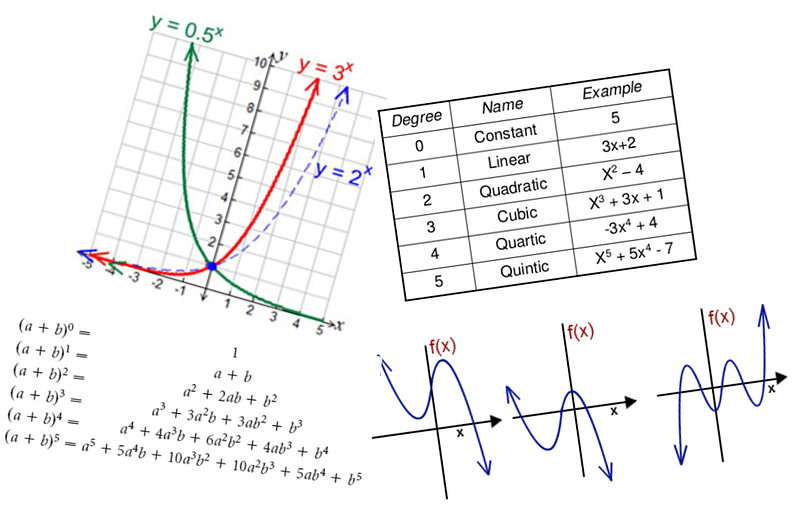What: Starting from absolute basic stuff like the equation of a line to binomial theorem and its properties.

• Logarithm, exponential, polynomial functions, rational numbers.
• Basic geometry and theorems, trigonometric identities.
• Real and complex numbers and basic properties.
• Series, sums, and inequalities.
• Graphing and plotting, Cartesian and polar co-ordinate systems, conic sections.

One (or two) example(s) where you might use it: If you want to understand how a search runs faster on a million item database after you sorted it, you will come across the concept of binary search. To understand the dynamics of it, logarithms and recurrence equations need to be understood. Or, if you want to analyze a time series you may come across concepts like periodic functionsand exponential decay.

Where do you learn:

Statistics:What: Absolute must-know to grow as a data scientist. The importance of having a solid grasp over essential concepts of statistics and probability cannot be overstated in a discussion about data science. Many practitioners in the field actually call classical (non neural network) machine learning nothing but statistical learning. The subject is vast and endless, and therefore focused planning is critical to cover most essential concepts.

• Data summaries and descriptive statistics, central tendency, variance, covariance, correlation,
• Basic probability: basic idea, expectation, probability calculus, Bayes theorem, conditional probability,
• Probability distribution functions — uniform, normal, binomial, chi-square, student’s t-distribution, Central limit theorem,
• Sampling, measurement, error, random number generation,
• Hypothesis testing, A/B testing, confidence intervals, p-values,
• ANOVA, t-test
• Linear regression, regularization

One (or two) example(s) where you might use it: In interviews. Trust me. As a prospective data scientist, if you can master all of the concepts mentioned above, you will impress the other side of the table really fast. And you will use some concept or other pretty much every day of your job as data scientist.

Where do you learn:

Linear Algebra: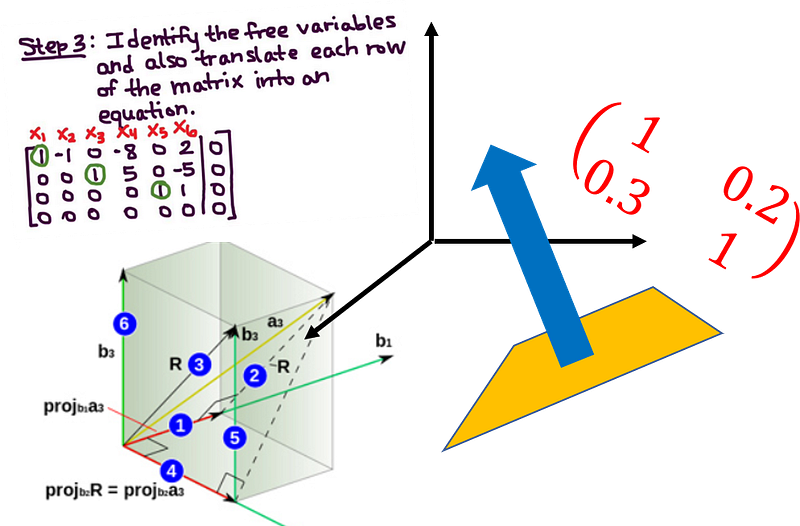What: Friend suggestion on Facebook. Song recommendation in Spotify. Transferring your selfie to a portrait drawing Salvador Dali style using Deep Transfer learning. What is common? Matrices and matrix algebra in all of them. This is an essential branch of mathematics to study for understanding how most machine learning algorithms work on a stream of data to create insight. Here are the essential topics to learn,

• Basic properties of matrix and vectors — scalar multiplication, linear transformation, transpose, conjugate, rank, determinant,
• Inner and outer products, matrix multiplication rule and various algorithms, matrix inverse,
• Special matrices — square matrix, identity matrix, triangular matrix, idea about sparse and dense matrix, unit vectors, symmetric matrix, Hermitian, skew-Hermitian and unitary matrices,
• Matrix factorization concept/LU decomposition, Gaussian/Gauss-Jordan elimination, solving Ax=b linear system of equation,
• Vector space, basis, span, orthogonality, orthonormality, linear least square,
• Eigenvalues, eigenvectors, and diagonalization, singular value decomposition (SVD)

One (or two) example(s) where you might use it: If you have used a dimensionality reduction technique Principal Component Analysis (PCA), then you have likely used the singular value decomposition to achieve a compact dimension representation of your data set with fewer parameters. All neural network algorithms use linear algebra techniques to represent and process the network structures and learning operations.

Where do you learn:

Calculus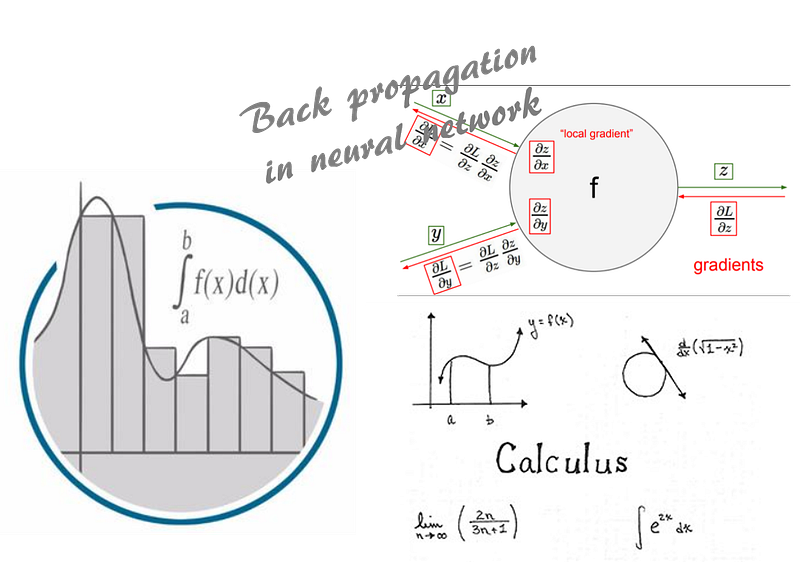What: The original maverick is back! Whether you loved it or hated it during college days, the fact is that the concept and application of calculus pops up in numerous places in the field of data science or machine learning. It lurks behind the simple looking analytical solution of ordinary least square problem in linear regression, or it is embedded in every back-propagation your neural network makes to learn a new pattern. It is an extremely valuable skill to add to your repertoire. Here are the topics to learn,

• Functions of single variable, limit, continuity and differentiability,
• Mean value theorems, indeterminate forms and L’Hospital rule,
• Maxima and minima,
• Product and chain rule,
• Taylor’s series, infinite series summation/integration concepts
• Fundamental and mean value-theorems of integral calculus, evaluation of definite and improper integrals,
• Beta and Gamma functions,
• Functions of multiple variables, limit, continuity, partial derivatives,
• Basics of ordinary and partial differential equations (not too advanced)

One (or two) example(s) where you might use it: Ever wondered how exactly a logistic regression algorithm is implemented. There is a high chance it is using a method called ‘Gradient descent’ to find the minimum loss function. To understand how this is working, you need to use concepts from calculus — gradient, derivatives, limits, and chain rule.

Where do you learn:

Discrete Math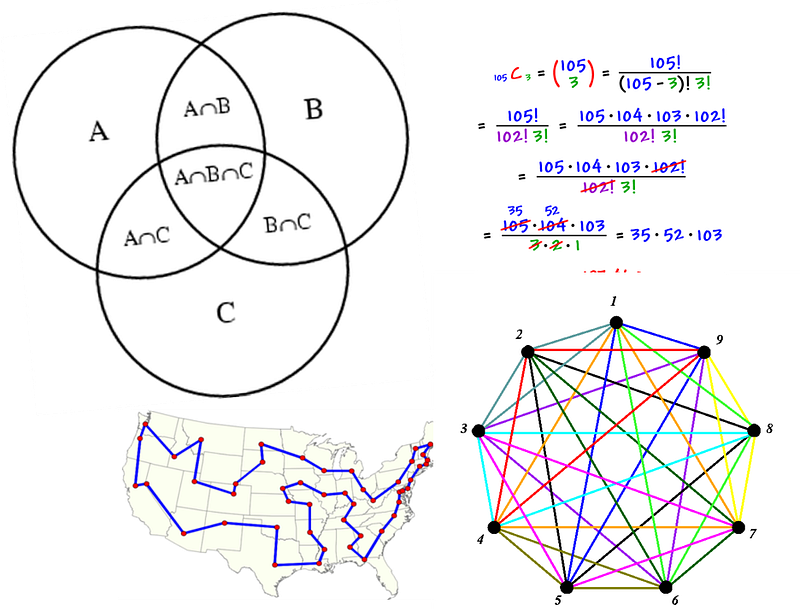What: This is often a less discussed topic in the scheme of “Math for Data Science” but the fact is that all modern data science is done with the help of computational systems and discrete math is at the heart of such systems. A refresher in discrete math will imbue the learner with concepts critical to her daily use of algorithms and data structures in analytics project. Some key topics to learn here,

• Sets, subsets, power sets
• Counting functions, combinatorics, countability
• Basic Proof Techniques — induction, proof by contradiction
• Basics of inductive, deductive, and propositional logic
• Basic data structures- stacks, queues, graphs, arrays, hash tables, trees
• Graph properties — connected components, degree, maximum flow/minimum cut concepts, graph coloring
• Recurrence relations and equations
• Growth of functions and O(n) notation concept

One (or two) example(s) where you might use it: In any social network analysis, you need to know properties of graph and fast algorithm to search and traverse the network. In any choice of algorithm you need to understand the time and space complexity i.e. how the running time and space requirement grows with input data size, by using O(n) (Big-Oh) notation.

Where do you learn:

Optimization, operation research topics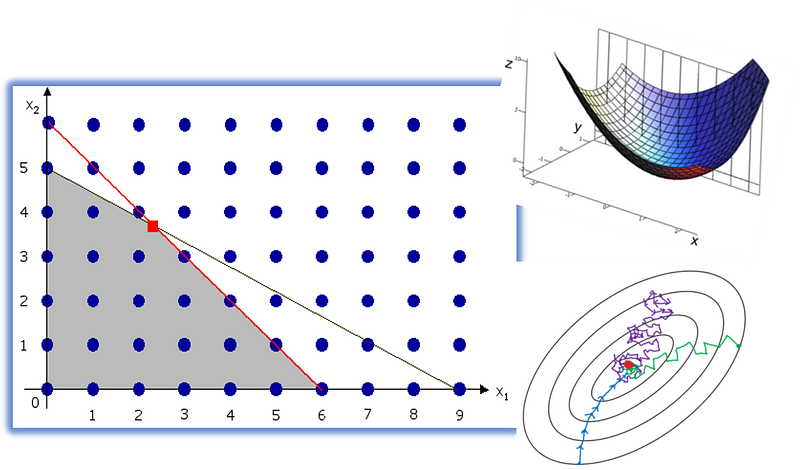What: These topics are little different from the traditional discourse in applied mathematics as they are mostly relevant and most widely used in specialized fields of study — theoretical computer science, control theory, or operation research. However, a basic understanding of these powerful techniques can be immensely fruitful in the practice of machine learning. Virtually every machine learning algorithm/technique aims to minimize some kind of estimation error subject to various constraints. That, right there, is an optimization problem. Topics to learn,

• Basics of optimization —how to formulate the problem
• Maxima, minima, convex function, global solution
• Linear programming, simplex algorithm
• Integer programming
• Constraint programming, knapsack problem

One (or two) example(s) where you might use it: Simple linear regression problems using least-square loss function often have a exact analytical solution. But logistic regression problems don’t. To understand the reason, you need to know the concept of convexity in optimization. This line of investigation will also illuminate why we have to remain satisfied with ‘approximate’ solutions in most machine learning problems. That’s a powerful truth to know deeply about.

Where do you learn:

### Some parting words

Do not need to be feel scared or lost. These are lot of things to learn and master, especially if you are not practicing them on a regular basis. But there are excellent resources online including wonderful videos. With some time and effort, you can make your own curated list of learning resource according to your personal need and level of comfort.

But you can be assured that, after refreshing these topics (many of which you may have studied in your undergraduate), and learning new concepts, you will feel so empowered that you will definitely start to hear the hidden music in your daily data analysis/machine learning projects. And that’s a big leap towards becoming a data scientist…

If you have any questions or ideas to share, please contact the author at tirthajyoti[AT]gmail.com. Also, you can check author’s GitHub repositories for other fun code snippets in Python, R, or MATLAB and machine learning resources. If you are, like me, passionate about machine learning/data science, please feel free to add me on LinkedIn or follow me on Twitter.

Bio: Tirthajyoti Sarkar is a semiconductor technologist, machine learning/data science zealot, Ph.D. in EE, blogger and writer.

Original. Reposted with permission.

Related:

By subscribing you accept KDnuggets Privacy Policy

By subscribing you accept KDnuggets Privacy Policy

By subscribing you accept KDnuggets Privacy Policy